# Filter or Extract for Multiple OR Criteria

If you are an valid MS Excel user, you have probably come across a situation where you wanted to filter the data in a separate table with specific criteria. You could do this task manually, which is also acceptable when dealing with a few data items. But if you got an assignment to filter out multiple items from a table consisting of a lot of data along with certain criteria, then doing these kinds of tasks manually would definitely be a stupid decision because this would not only waste your precious time, but you would also get tired of it and won’t complete your task on time.

But don’t be worry about it; for getting out of this fix and filtering out multiple data with specific criteria, all you have to do is read this article carefully.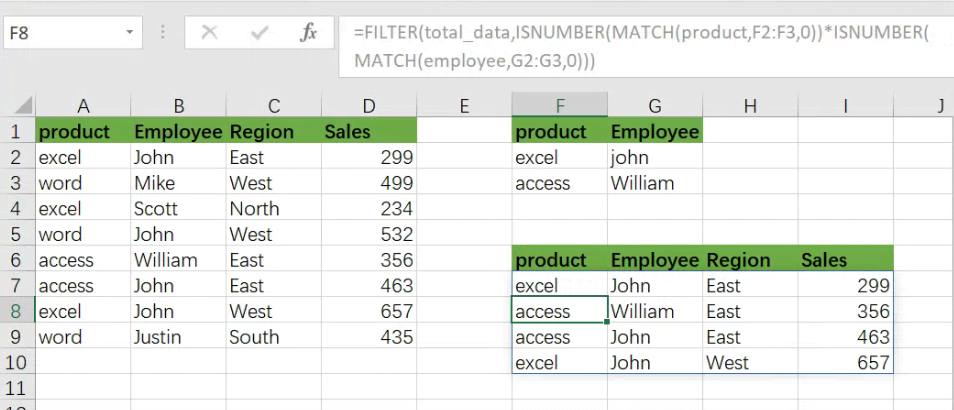So let’s dive into it.

## General formula:

The formula below would help you filter out multiple data with specific criteria within a few seconds.

As we have altered the following formula according to the example which we would discuss in this article to understand that how this formula works and how to use this formula:

` =FILTER(total_data,ISNUMBER(MATCH(product,F2:F3,0))*ISNUMBER(MATCH(employee,G2:G3,0)))`In the formula stated above, we are using the filter function along with the Match function, where ranges are specified for products(A2:A9), employee (B2:B9), and regions (C2:C9).

This formula produces information when the product is “excel” or “access”, AND the employee are “john” or “William”.

## Syntax Explanation:

Before we dive into the formula for getting the job done effectively, we need to understand each syntax so that we can know how each syntax helps to Filter with multiple OR criteria :

• `Filter`: This tool helps to narrow down or filter out a variety of data depending on user-defined criteria.
• `Comma symbol` (,): In Excel, this symbol functions as a separator and plays a vital role in separating a list of values.
• `Parenthesis` (): Its primary role is to group and separate elements.
• `ISNUMBER`: The ISNUMBER function determines if a value in a cell or a value derived from another formula is a number. ISNUMBER returns either “`true`” or “`false`.”
• `MATCH`: The MATCH function looks for a given item in a range of cells and returns the item’s relative location in the range.

## Let’s See How This Formula Works:

Criteria for filtering out multiple data are entered in the range F2:G3 in this example. The formula’s rationale is as follows: the product is “excel” or “access”, AND the employee are “john” or “William”.

This formula’s filtering logic (the include parameter) is used with the ISNUMBER and MATCH functions and boolean logic in an array operation.

MATCH is set up “backward,” using lookup values from the data and criteria for the lookup array. For example, the first requirement is that the product be either “excel” or “access”. MATCH is configured as follows to apply this condition:

`=MATCH(product,F2:F3,0) // look for excel product`

As in the example, there are 8 values in the data; that’s why we get an array with 8 values that looks like the following: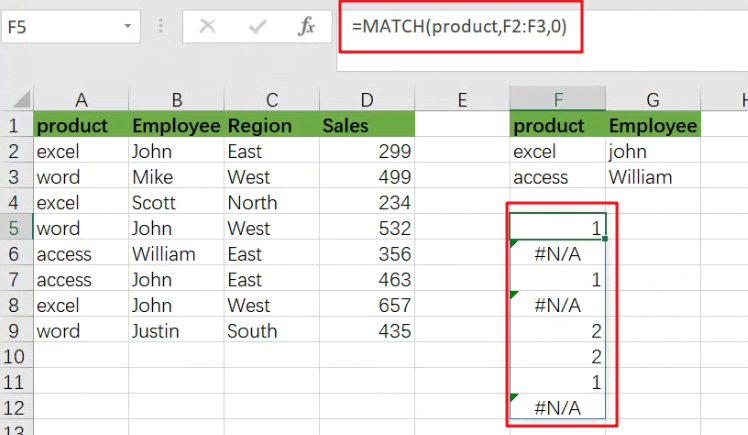The above array would include `#N/A` errors (no match) or numbers (match). The numbers on the notice refer to either “excel” or “access” products. To turn this array into TRUE and FALSE values, the MATCH function is wrapped in the ISNUMBER function:

`=ISNUMBER(MATCH(product,F2:F3,0))`

which results in an array like the following one: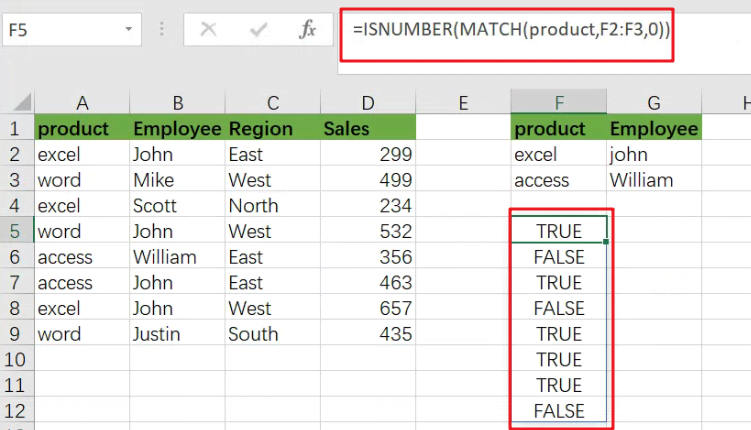TRUE values in this array match a “excel” or “access”.

The exclusive formula has two expressions similar to the ones used for the FILTER function’s include argument.

Following the evaluation of MATCH and ISNUMBER, we get two arrays containing TRUE and FALSE values. The arithmetic action of multiplying these arrays together converts the TRUE and FALSE values to 1s and 0s.

Following the laws of boolean arithmetic, the outcome is a single array which is stated as follows: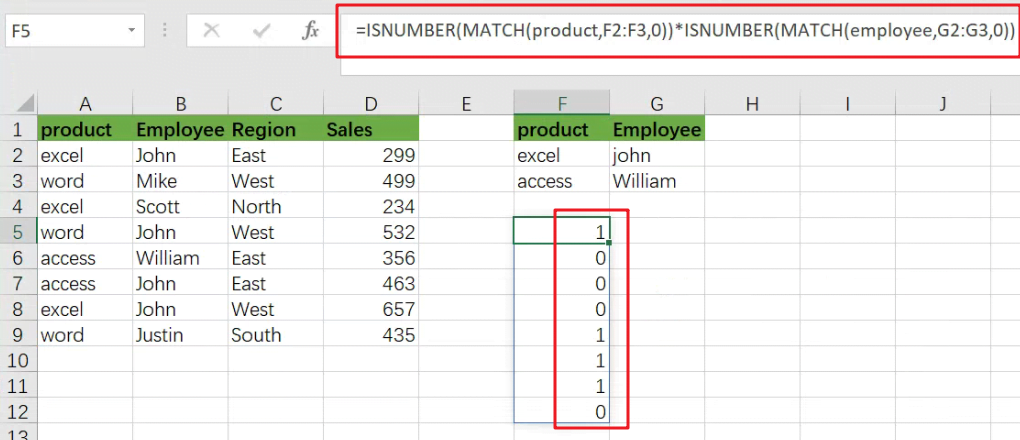which is sent as an argument to the FILTER function like the following:

`=FILTER(B5:D16,{1;0;0;0;0;1;0;0;0;0;0;1})`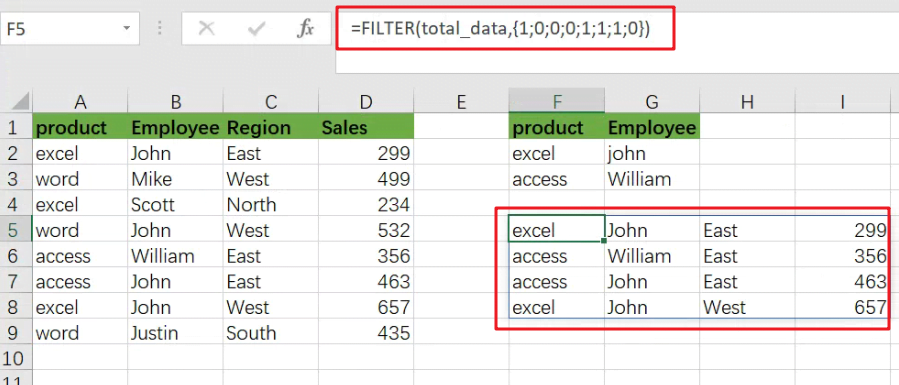### Related Functions

• Excel ISNUMBER function
The Excel ISNUMBER function returns TRUE if the value in a cell is a numeric value, otherwise it will return FALSE.The syntax of the ISNUMBER function is as below:= ISNUMBER (value)…
• Excel Filter function
The FILTER function extracts matched records from a collection of data using one or more logical checks. The include argument specifies logical tests, which might encompass a wide variety of formula conditions.==FILTER(array,include,[if empty])…
• Excel MATCH  function
The Excel MATCH function search a value in an array and returns the position of that item.The MATCH function is a build-in function in Microsoft Excel and it is categorized as a Lookup and Reference Function.The syntax of the MATCH function is as below:= MATCH  (lookup_value, lookup_array, [match_type])….
Related Posts

Break ties with helper COUNTIF and column

Suppose you got a task to adjust the values that contain the ties; what would be your first attempt to break the ties of the given value? If you are wondering about doing this task manually, let me add that ...

Find the Closest Data to the Data Provided in Excel

In our daily work, we may encounter such an issue that to find the closest value to a certain value. In fact, Excel internal functions can help us solve this problem. In today’s article, we will show you how to ...

Abbreviate Names Or Words in Excel

As an MS Excel user, you might have come across a task where you need to abbreviate different names or words, and there are also possibilities that you might have done this task manually by assuming that there isn't any ...

Convert State Names To Abbreviations

Assume that you got a task to convert the full state's name into the abbreviations in MS Excel, and for doing this task, you might choose to do it manually, which is an acceptable way only if you don't have ...

Filter or Remove Columns

Assume that in MS Excel, you have a table consisting of a few columns consisting of few values, and you want to filter to remove the specified columns from the table. You might take it easy and would prefer to ...

Filter Multiple Values

In Excel, you can easily filter a table to display only the rows that meet your criteria. This is a quick way to find the information you need without scrolling through all the data. In this post, we'll show you ...

Excel XLOOKUP Function

Excel XLOOKUP Function was added into Excel as a beta feature in August 2019 and is now accessible exclusively in Microsoft 365. (as of July 2021). However, if you fall into this category and often deal with big sets of ...

Extract or Filter Top n values

You might have been through this kind of situation where you need to filter out the top n values from the list having few values, and I am also pretty sure about it that you might have chosen to do ...

Extract Unique Items From A List

This post will guide you how to extract unique itmes from a given list in Microsoft Excel. How to create a newly formula to get unique values from a range cells in Excel. The unique list of items is the ...

Extract all the matches with helper Column

With Excel's powerful functions IF, INDEX, and MATCH, we can find exactly what you're looking for with a few clicks of the mouse. This step-by-step tutorial will show how easy it is to extract data using these tools and more! ...

Sidebar